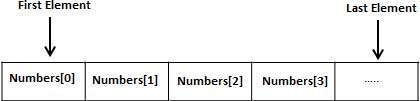Objective-C Arrays

Objective-C programming language provides a data structure called the array, which can store a fixed-size sequential collection of elements of the same type. An array is used to store a collection of data, but it is often more useful to think of an array as a collection of variables of the same type.

Instead of declaring individual variables, such as number0, number1, ..., and number99, you declare one array variable such as numbers and use numbers, numbers, and ..., numbers to represent individual variables. A specific element in an array is accessed by an index.

All arrays consist of contiguous memory locations. The lowest address corresponds to the first element and the highest address to the last element.Declaring Arrays

To declare an array in Objective-C, a programmer specifies the type of the elements and the number of elements required by an array as follows −

type arrayName [ arraySize ];

This is called a single-dimensional array. The arraySize must be an integer constant greater than zero and type can be any valid Objective-C data type. For example, to declare a 10-element array called balance of type double, use this statement −

double balance;

Now, balance is a variable array, which is sufficient to hold up to 10 double numbers.

Initializing Arrays

You can initialize an array in Objective-C either one by one or using a single statement as follows −

double balance = {1000.0, 2.0, 3.4, 17.0, 50.0};

The number of values between braces { } can not be larger than the number of elements that we declare for the array between square brackets [ ]. Following is an example to assign a single element of the array −

If you omit the size of the array, an array just big enough to hold the initialization is created. Therefore, if you write −

double balance[] = {1000.0, 2.0, 3.4, 17.0, 50.0};

You will create exactly the same array as you did in the previous example.

balance = 50.0;

The above statement assigns element number 5th in the array a value of 50.0. Array with 4th index will be 5th, i.e., last element because all arrays have 0 as the index of their first element which is also called base index. Following is the pictorial representation of the same array we discussed above −Accessing Array Elements

An element is accessed by indexing the array name. This is done by placing the index of the element within square brackets after the name of the array. For example −

double salary = balance;

The above statement will take 10th element from the array and assign the value to salary variable. Following is an example, which will use all the above mentioned three concepts viz. declaration, assignment and accessing arrays −

#import <Foundation/Foundation.h>

int main () {
int n[ 10 ];   /* n is an array of 10 integers */
int i,j;

/* initialize elements of array n to 0 */
for ( i = 0; i < 10; i++ ) {
n[ i ] = i + 100;    /* set element at location i to i + 100 */
}

/* output each array element's value */
for (j = 0; j < 10; j++ ) {
NSLog(@"Element[%d] = %d\n", j, n[j] );
}

return 0;
}

When the above code is compiled and executed, it produces the following result −

2013-09-14 01:24:06.669 demo Element = 100
2013-09-14 01:24:06.669 demo Element = 101
2013-09-14 01:24:06.669 demo Element = 102
2013-09-14 01:24:06.669 demo Element = 103
2013-09-14 01:24:06.669 demo Element = 104
2013-09-14 01:24:06.669 demo Element = 105
2013-09-14 01:24:06.669 demo Element = 106
2013-09-14 01:24:06.669 demo Element = 107
2013-09-14 01:24:06.669 demo Element = 108
2013-09-14 01:24:06.669 demo Element = 109

Objective-C Arrays in Detail

Arrays are important to Objective-C and need lots of more details. There are following few important concepts related to array which should be clear to a Objective-C programmer −

Sr.No. Concept & Description
1 Multi-dimensional arrays

Objective-C supports multidimensional arrays. The simplest form of the multidimensional array is the two-dimensional array.

2 Passing arrays to functions

You can pass to the function a pointer to an array by specifying the array's name without an index.

3 Return array from a function

Objective-C allows a function to return an array.

4 Pointer to an array

You can generate a pointer to the first element of an array by simply specifying the array name, without any index.

Useful Video Courses

Video

Understanding S.M.A.R.T. Objectives

18 Lectures 1 hours